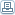# An Insiders Secret About Fibonacci Time Analysis

 By: Delija CrnovrsaninIf you have at least a few months that Forex came into your life you have surely heard of Fibonacci levels in Forex charts. But what is Fibonacci? Fibonacci sequence is a series of numbers. Every number is being produced by adding the last Fibonacci number to the previous. The first numbers of Fibonacci sequence are 1,2,3,5,8,13,21,34,55,....etc But what has Fibonacci sequence to do with Forex Trading? IF you divide two sequential numbers you get the result 1,618. The square of 1,618 is 1,27. The inverse number of 1,618 is 0,618. The inverse of 1,27 is 0,786. These numbers are called Fibonacci numbers because they result from Fibonacci sequence number's analogies. The 1,618 number was called 'Golden Mean' by ancient Greeks and other ancient cultures. They called it so because they observed that this number is found everywhere in nature. The result of creations, living organisms to space galaxies, that have this number embedded is symmetry. But enough with maths and science! Let's see the use of Fibonacci numbers in trading. Since the beginning of investment industry, traders have noticed that prices tend to change direction in levels that are very close to these numbers I mentioned above. For example in an unptrend the prices will go up and then swing down to a level that is a Fibonacci number before continuing the uptrend. These levels are called Fibonacci Retracement levels. The most common Fibonacci levels in Forex market are 0.382, 0.5, 0.618 and 0.786. Nobody knows why prices tend swing in these Fibonacci levels. And nobody knows at which exact Fibonacci level will the price change direction in advance. How could you use this knowledge to improve your trading? Well, you should know that prices tend to reverse at Fibonacci retracement levels. A lot of novice traders use the exact point of a fibonacci retracement level e.g. 0.618 as a trade entry. Experienced traders know this fact and wait for other traders to get their stop loss hit and then enter the market. Fibonacci retracement levels should be used as an indication of entry and not as the exact point of entry. Moreover the bulk of traders use 0,618 and 0.386 retracement levels. Experienced traders know this tendency and wait for other retracement levels not widely used like 0.786 or 0.707 in order to enter a trade. Use these Fibonacci retracements as well. Make the difference! But how would you know at which Fibonacci retracement level will the price change direction? Fibonacci retracements, like other technical indicators are more valid when they are calculated for a greater time value. Do not pick minor swings to calculate Fibonacci retracements. Pick greater price swings instead. Moreover, a Fibonacci level becomes more valid when it coincides with another technical indicators such as trendline resistance or support, MACD or RSI divergence and so on. The most valid retracement level should be choosen keeping in mind that further confirmation from other technical indicators should be taken into account. You wouldn't like to put your money on risk with only one reason, would you? So choose the Fibonacci retracement level that coincides with other reverse signals. Last of all let's see the use of Fibonacci numbers in trading time analysis. Pick a significant hi or a low in a daily chart. Then calculate trading days (excluding weekends) from that point and on using Fibonacci sequence. You would have the first, the second, the third, the fifth the eighth trading day and so on. Watch that in trading days that are Fibonacci numbers, prices tend to reverse direction! Isn't it amazing? Add this tool to your chart analysis and you wont lose! After all these years of trading experience and research I have found that Forex charts iclude some special price patterns created by price swings. These patterns are formed under certain price relations between their swings. My trade system will provide you with low risk and hi reward trading entries. Think smart. Learn more: